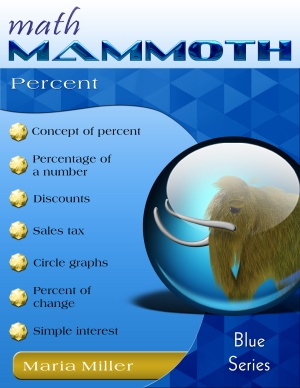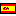^

# Free video lessons on percents and percentages (6th-7th grades)

Find here free math videos on percent-related topics: what is percent, percentage of a number, discouns, sales tax, and percent of change. These videos are meant equally for students, teachers, and parents.

The videos match the lessons in my book Math Mammoth Percent (Blue Series book), or the lessons in chapter 5 of Grade 6-A (Light Blue series), or chapter 7 in Grade 7-B (Light Blue series). In any of those books, you will get MANY more practice exercises, word problems, and puzzles than what are shown in the videos, and also some lessons that are not in covered in this set of videos.

## Percent

What is percent? The main point... percentages are just FRACTIONS. "Five per cent" literally means "five per 100", or 5 / 100 — five hundredths. Of course we can also write this as the decimal 0.05.

I this lesson, we first practice the concept of percent and write percents as fractions and as decimals and vice versa. Then we also convert fractions to percents.

## Percent of a Number

Find how to solve various percentages (percent of a number or quantity) using mental math. First, find 1% or 10% of the number, then you can find any percentage of the number.

In the second part, I solve two simple word problems involving percentages, using mental math. One is about taxes and the other is about discounts.

## Percent of a number using decimals

I show how to find a percent of a number, such as 8% of 5,000, using decimals. We change the word "of" into multiplication and the percent into a decimal. Then you just multiply using a calculator, mental math, or paper & pencil.

## What percentage / How many percent?

To answer these kinds of questions (How many percent?/What percentage?), first write the PART being asked. You can replace the question in the problem with, "What part...?" Write that as a fraction. THEN, simply convert that fraction into a decimal and on into a percent.

## How to solve basic percent word problems

These two video lessons give examples and full solutions to several basic percentage word problems, most of which involve the concept of PART/TOTAL = PERCENTAGE.

First I present three problems that all involve a discount. The first asks for the discount percentage, the second for the discount price, and the third for the original price. Then I also solve a problem where the original price and a percentage price increase are given, and the new price is asked.

In the second video, we first find what percentage the area of one triangle is of the are of the other. Of course, to find the percentage, we simply write the fraction PART/TOTAL, and then convert that to a percentage. Then we solve a problem involving an overtime pay that is 160% of the normal.

The last problem states, "3.1 is 14% of what number?" — a typical algebra textbook problem. Essentially, we need to find the TOTAL when the part and the percentage are known. One way to do that is to use the little "formula" PART/TOTAL = PERCENTAGE. In this case, we get 3.1/x = 0.14 or 3.1/x = 14/100, and it's easy to solve the latter equation with cross-multiplying.

## How to calculate sales tax

I show how to calculate the final price when you know the sales tax percentage. I show a few examples of using mental math and a few to be done with a calculator. I also show how to make this into a single calculation instead of first calculating the tax and then adding (which is two calculations).

## Percent of Change

This is a beginner lesson explaining how to calculate the percentage of change. We simply find the actual DIFFERENCE between the original and the new quantities, and divide that by the original quantity. In other words, we write the fraction DIFFERENCE/ORIGINAL, or compare the actual change to the original amount. Then all you do is write that fraction as a percentage (possibly first changing it to a decimal with a calculator, and then from a decimal to a percentage).

## Percent of Change - Applications

I solve two word problems that involve percentage change - one having to do with percentage increase in area, and another with two separate discounts.

## Relative difference or percentage difference — comparing values using a percentage

All we need to do is write the fraction DIFFERENCE/REFERENCE and then convert that into a percentage. The "difference" is the difference between the two values we are comparing. The reference value could be one of the two values, if it is clear that we are comparing one item to the other. For example, if the question is how many percent taller is the oak tree than a birch tree, then the comparison is TO the birch tree so its height is the reference value. If it's not clear which of the two is the "reference item", then you can use the average of the two values as the reference value. However, this last situation is not deal with in the video.

The videos match the lessons in my book Math Mammoth Percent (Blue Series book), or the lessons in chapter 5 of Grade 6-A (Light Blue series), or chapter 7 in Grade 7-B (Light Blue series). In any of those books, you will get MANY more practice exercises, word problems, and puzzles than what are shown in the videos, and also some lessons that are not in covered in this set of videos.80 pages (includes answers) Math Mammoth Percent PDF download \$3.50 Add to cartEste libro en español

By Maria Miller

WAIT!

Receive my monthly collection of math tips & resources directly in your inbox — and get a FREE Math Mammoth book!You can unsubscribe at any time.

### Math Mammoth Tour

Confused about the different options? Take a virtual email tour around Math Mammoth! You'll receive:

An initial email to download your GIFT of over 400 free worksheets and sample pages from my books. Six other "TOURSTOP" emails that explain the important things and commonly asked questions concerning Math Mammoth curriculum. (Find out the differences between all these different-colored series!)

This way, you'll have time to digest the information over one or two weeks, plus an opportunity to ask me personally about the curriculum.
A monthly collection of math teaching tips & Math Mammoth updates (unsubscribe any time)### "Mini" Math Teaching Course

This is a little "virtual" 2-week course, where you will receive emails on important topics on teaching math, including:

- How to help a student who is behind
- Troubles with word problems
- Teaching multiplication tables
- Why fractions are so difficult
- The value of mistakes
- Should you use timed tests
- And more!

A GIFT of over 400 free worksheets and sample pages from my books right in the very beginning.A monthly collection of math teaching tips & Math Mammoth updates (unsubscribe any time)
Enter your email to receive math teaching tips, resources, Math Mammoth news & sales, humor, and more! I tend to send out these tips about once monthly, near the beginning of the month, but occasionally you may hear from me twice per month (and sometimes less often).• A GIFT of over 400 free worksheets and sample pages from my books.